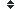be parallel (3 synonym found)

### Sort be parallel by:    Alphabetically| Length| Random | DefinitionDefinition of be parallel:

(adj) : of two or more (straight) lines, (flat) surfaces etc: Equally distant from one another at all points; parallel to: Of one thing, relative to another: Equally distant from at all points
"the horizontal lines on my notebook paper are parallel."
(n) : one of a set of parallel lines; a line of latitude
"the 31st parallel passes through the center of my town."
(v) : to construct something parallel to something else; of a path etc: To be parallel to something else
History
This word has been
viewed 392 times.

Definition of be parallel:

(adj) : of two or more (straight) lines, (flat) surfaces etc: Equally distant from one another at all points; parallel to: Of one thing, relative to another: Equally distant from at all points
"the horizontal lines on my notebook paper are parallel."
(n) : one of a set of parallel lines; a line of latitude
"the 31st parallel passes through the center of my town."
(v) : to construct something parallel to something else; of a path etc: To be parallel to something else